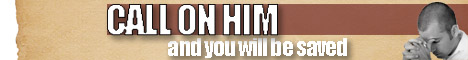Note:  Do not rely on this information. It is very old.

# Formula

Formula, in general Physics, is an equation which will determine the magnitude of some physical quantity, when proper numerical data are known and applied. Similarly, in pure mathematics, a formula means the equation connecting a variable quantity with those upon which its value depends.